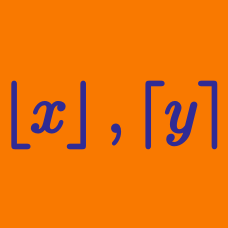Algebra

# Ceiling Function

Which of the following statements is/are true?

I. $$\lceil x+n\rceil=\lceil x\rceil+n$$ for any real $$x$$ and any integer $$n.$$
II. $$\lceil x\rceil+\lceil-x\rceil=1$$ if and only if $$x$$ is not an integer.
III. $$\lceil x\rceil+\lceil y\rceil\le\lceil x+y\rceil\le\lceil x\rceil+\lceil y\rceil+1$$ for any real $$x$$ and $$y.$$

What is the solution set to $$\lceil\frac{x}{3}-8\rceil=10?$$

What is $$\lceil\log_{5}59\rceil?$$

How many integers $$x$$ satisfy the equation $\lceil\log_{3}x\rceil=4?$

What is $$\lceil-\pi\rceil?$$

×